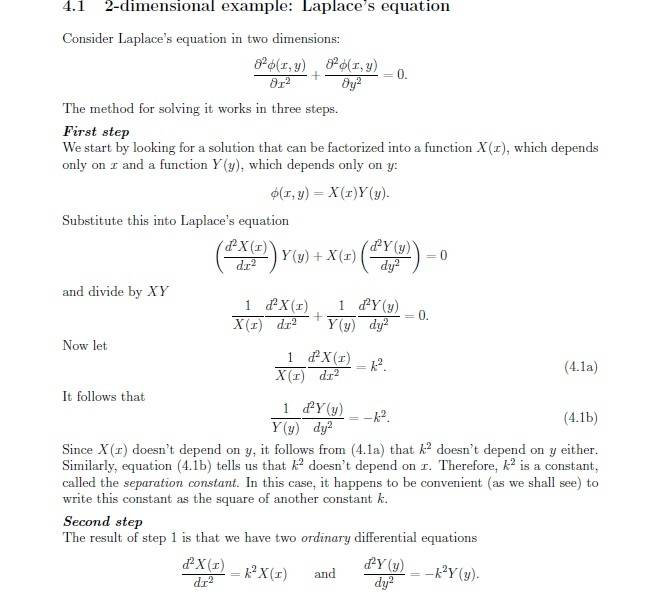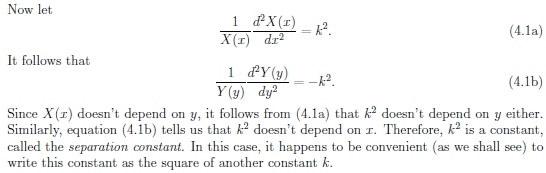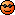# Solve PDE by separation of variables

## Homework Statement

Solve ∇2T(x, y) = 0
with boundary conditions
T(0, y) = T(L, y) = T0
T(x, L/2) = T(x, -L/2) = T0 + T1sin(πx/L)

## The Attempt at a Solution

Set T(x, y) = X(x)Y(y)

Then ∇2T(x,y) = (∂2X/∂x2) Y + (∂2Y/∂y2) X = 0

Rearrange to find two separate ODEs:

d2X/dx2 = k2X
d2Y/dy2 = -k2Y

Two solutions:

X(x) = Aekx + Be-kx
Y(x) = Ceiky + De-iky

Thus

T(x, y) = (Aekx + Be-kx)(Ceiky + De-iky)

Boundary conditions:

T(0, y) = (A+B)(Ceiky + De-iky) = T0
T(L, y) = (AekL + Be-kL)(Ceiky + De-iky) = T0

Equate the two and rearrange to find:

B = AekL

Next boundary condition:

T(x, L/2) = (Aekx + Be-kx)(CeikL/2 + De-ikL/2) = T0 + T1sin(πx/L)
T(x, -L/2) = (Aekx + Be-kx)(Ce-ikL/2 + DeikL/2) = T0 + T1sin(πx/L)

Equate the two and rearrange to find:

C=D

Thus:

T(x, y) = AC(ekx + ekLe-kx)(eiky + e-iky)

Returning to first boundary conditions:

T(0, y) = AC(1 + ekL)(eiky + e-iky) = T0

True for all y, so set y=0, then can find:

AC = T0/2(1+ekL)

So now:

T(x, y) = (T0/2(1+ekL))(ekx + ekLe-kx)(eiky + e-iky)

Returning to second boundary condition:

T(x, L/2) = (T0/2(1+ekL))(ekx + ekLe-kx)(eikL/2 + e-ikL/2) = T0 + T1sin(πx/L)

True for all x, so set x=0:

T(0, L/2) = (T0/2(1+ekL))(1 + ekL)(eikL/2 + e-ikL/2) = T0
Reduces to:
(eikL/2 + e-ikL/2) = 2

Which solves to give:

k = 2πn/L , for n∈ℤ

Thus:

T(x, y) = (T0/2(1+e2πn))(e2πnx/L + e2πne-2πnx/L)(ei2πny/L + e-i2πny/L)

But when I check this back for ∇2T(x, y) = 0 it doesn't work.

I think the problem is where I make the "same for all x so set x=0" assumptions..?

But if I don't make those assumptions I can't find AC or k.

It's all rather confusing..!

Then ∇2T(x,y) = (∂2X/∂x2) Y + (∂2Y/∂y2) X = 0

Rearrange to find two separate ODEs:

d2X/dx2 = k2X
d2Y/dy2 = -k2Y

I think there is problem of assuming such separate forms
you are solving a Laplace equation which is often used in physics and there are some conditions on first derivative of the function T
The Laplace equation for T implies that the integrability condition for T is satisfied: T must be analytic on the plane The solutions of Laplace's equation are the harmonic functions, which are important in many fields of science
These equations have the solutions
If k is not zero
Y cos kx or sin kx
http://web.mit.edu/6.013_book/www/chapter5/Images/eqn-5.29.gif
If k = 0, the solutions degenerate into

http://web.mit.edu/6.013_book/www/chapter5/Images/eqn-5.30.gif

http://web.mit.edu/6.013_book/www/chapter5/Images/eqn-5.31.gif
pl. look up a ref. say http://web.mit.edu/6.013_book/www/chapter5/5.4.html

Mark44
Mentor
Your title is misleading. You aren't solving an ODE (ordinary DE) -- you're solving a PDE (partial DE). Let me know and I can change it.

## Homework Statement

Solve ∇2T(x, y) = 0
with boundary conditions
T(0, y) = T(L, y) = T0
T(x, L/2) = T(x, -L/2) = T0 + T1sin(πx/L)

## The Attempt at a Solution

Set T(x, y) = X(x)Y(y)
You're not setting T(x, y) -- you're assuming that T(x, y) can be written as the product of a function of x alone and a function of y alone.
sa1988 said:
Then ∇2T(x,y) = (∂2X/∂x2) Y + (∂2Y/∂y2) X = 0

Rearrange to find two separate ODEs:

d2X/dx2 = k2X
d2Y/dy2 = -k2Y
How did you get the two equations above?
sa1988 said:
Two solutions:

X(x) = Aekx + Be-kx
Y(x) = Ceiky + De-iky

Thus

T(x, y) = (Aekx + Be-kx)(Ceiky + De-iky)

Boundary conditions:

T(0, y) = (A+B)(Ceiky + De-iky) = T0
T(L, y) = (AekL + Be-kL)(Ceiky + De-iky) = T0

Equate the two and rearrange to find:

B = AekL

Next boundary condition:

T(x, L/2) = (Aekx + Be-kx)(CeikL/2 + De-ikL/2) = T0 + T1sin(πx/L)
T(x, -L/2) = (Aekx + Be-kx)(Ce-ikL/2 + DeikL/2) = T0 + T1sin(πx/L)

Equate the two and rearrange to find:

C=D

Thus:

T(x, y) = AC(ekx + ekLe-kx)(eiky + e-iky)

Returning to first boundary conditions:

T(0, y) = AC(1 + ekL)(eiky + e-iky) = T0

True for all y, so set y=0, then can find:

AC = T0/2(1+ekL)

So now:

T(x, y) = (T0/2(1+ekL))(ekx + ekLe-kx)(eiky + e-iky)

Returning to second boundary condition:

T(x, L/2) = (T0/2(1+ekL))(ekx + ekLe-kx)(eikL/2 + e-ikL/2) = T0 + T1sin(πx/L)

True for all x, so set x=0:

T(0, L/2) = (T0/2(1+ekL))(1 + ekL)(eikL/2 + e-ikL/2) = T0
Reduces to:
(eikL/2 + e-ikL/2) = 2

Which solves to give:

k = 2πn/L , for n∈ℤ

Thus:

T(x, y) = (T0/2(1+e2πn))(e2πnx/L + e2πne-2πnx/L)(ei2πny/L + e-i2πny/L)

But when I check this back for ∇2T(x, y) = 0 it doesn't work.

I think the problem is where I make the "same for all x so set x=0" assumptions..?

But if I don't make those assumptions I can't find AC or k.

It's all rather confusing..!

I think there is problem of assuming such separate forms
you are solving a Laplace equation which is often used in physics and there are some conditions on first derivative of the function T
The Laplace equation for T implies that the integrability condition for T is satisfied: T must be analytic on the plane The solutions of Laplace's equation are the harmonic functions, which are important in many fields of science
These equations have the solutions
If k is not zero
Y cos kx or sin kx
http://web.mit.edu/6.013_book/www/chapter5/Images/eqn-5.29.gif
If k = 0, the solutions degenerate into

http://web.mit.edu/6.013_book/www/chapter5/Images/eqn-5.30.gif

http://web.mit.edu/6.013_book/www/chapter5/Images/eqn-5.31.gif
pl. look up a ref. say http://web.mit.edu/6.013_book/www/chapter5/5.4.html

My solutions for X(x) and Y(y) are also harmonic. Unless I'm mistaken, I've just written them in exponential form.
https://en.wikipedia.org/wiki/Euler's_formula#Relationship_to_trigonometry

Your title is misleading. You aren't solving an ODE (ordinary DE) -- you're solving a PDE (partial DE). Let me know and I can change it.

Ah, my mistake. Please do change it!

You're not setting T(x, y) -- you're assuming that T(x, y) can be written as the product of a function of x alone and a function of y alone.

My mistake again. I assumed T(x, y) can be separated.

How did you get the two equations above?

Instead of a messy attempt at typing it out, here's a screen grab of my lecture notes which show the exact process I used:As far as I can tell, my method seems to be ok but I'm stumbling on the boundary conditions.

I've had a look at some other notes provided by the lecturer and at a guess I'd say my problem is that I need to find a way to lose the x-dependent and y-dependent terms when I work through the boundary conditions.

For example:

T(0, y) = AC(1+ekL)(eiky+e-iky) = T0

has a y-dependent term which needs removing before I can have a stab at finding the constants AC or k.

SammyS
Staff Emeritus
Homework Helper
Gold Member
Rearrange to find two separate ODEs:

d2X/dx2 = k2X
d2Y/dy2 = -k2Y

Two solutions:

X(x) = Aekx + Be-kx
Y(x) = Ceiky + De-iky
...

I see that your notes say:.
What is it that makes this convenient?

It seems to me that your choice of using the negative separation constant with Y rather than with X commits Y to being sinusoidal and X to be exponential.

Unless I'm misreading them, the boundary conditions suggest just the opposite assignment.

I see that your notes say:
View attachment 96290 .
What is it that makes this convenient?

It seems to me that your choice of using the negative separation constant with Y rather than with X commits Y to being sinusoidal and X to be exponential.

Unless I'm misreading them, the boundary conditions suggest just the opposite assignment.

I've just run it through with the differential equation's signs alternated as you suggested, and it still comes out with an almost identical dead end.

Only difference is I get an imaginary term. In my first attempt I get as far as:
B=AekL
C=D

In this attempt I've ended up with:
B=AeikL
C=D

It's all much the same reallyI'd have though the sign for the k2 term is largely irrelevant anyway as it's only there to pose as an arbitrary term in general solution.

LCKurtz
Homework Helper
Gold Member
I'm not going to try to rescue what has been done. Rather, I will outline what I believe to be a much better approach to working this problem. You have ##T_{xx} + T_{yy} = 0## with boundary conditions ##T(0,y)=T(L,y) = T_0##. Let's leave the other boundary condition for later. You don't want to do separation of variables with non-homogeneous BC's like that. You need to address the non-homogeneous aspect first. So let's make the substitution ##T(x,y) = u(x,y) + \Psi(x)## with the intention of letting the ##\Psi(x)## take care of the NH term. Plugging this equation into the above PDE and boundary condition gives:$$u_{xx}(x,y) + \Psi''(x) + u_{yy}(x,y) = 0$$ $$T(0,y)=u(0,y)+\Psi(0) = T_0,~~T(L,y) = u(L,y) + \Psi(L) = T_0$$
Now, if we can find a ##\Psi(x)## satisfying ##\Psi''(x)=0, ~\Psi(0) = \Psi(L) = T_0## (we can, it's trivially ##\Psi(x) \equiv T_0##) these two equations become$$u_{xx}(x,y) + u_{yy}(x,y) = 0$$ $$u(0,y)= 0,~~u(L,y) =0$$
This is the equation you want to separate variables on: ##u(x,y) = X(x)Y(y)##. In the usual way this leads to the two equations$$X''-\lambda X = 0,~ Y''+\lambda Y = 0$$ $$X(0) = X(L) = 0$$It's easy enough to check that ##\lambda = -\mu^2 < 0## gives nontrivial solutions for the ##X## equation:$$\mu_n = \frac{n\pi}{L},~~X_n(x) = \sin(\frac{n\pi x}{L})$$With this choice of ##\lambda## the corresponding solutions for the ##Y## equation are$$Y_n(y) = C_n\cosh(\frac{n\pi y }{L})+ D_n\sinh(\frac{n\pi y}{L})$$So our proposed solution for ##u(x,y)## is$$u(x,y) = \sum_{n=1}^\infty \sin(\frac{n\pi x}{L})\left( C_n\cosh(\frac{n\pi y }{L})+ D_n\sinh(\frac{n\pi y}{L})\right)$$and our proposed solution for ##T(x,y)## is$$T(x,y) = u(x,y) + \Psi(x) = T_0 +\sum_{n=1}^\infty \sin(\frac{n\pi x}{L})\left ( C_n\cosh(\frac{n\pi y }{L})+ D_n\sinh(\frac{n\pi y}{L})\right)$$At this point, it isn't difficult to figure out what the constants ##C_n,~D_n## need to be to get your final boundary condition$$T(x,\frac L 2)=T(x,-\frac L 2) = T_0 + T_1\sin(\frac {\pi x}{L})$$to work.

I will admit to showing my biases here. The use of complex exponentials just makes it hard to read and see what is going on, and the use of the sinh and cosh pair is also more natural than the exponential pair.

Last edited:
•sa1988
I'm not going to try to rescue what has been done. Rather, I will outline what I believe to be a much better approach to working this problem. You have ##T_{xx} + T_{yy} = 0## with boundary conditions ##T(0,y)=T(L,y) = T_0##. Let's leave the other boundary condition for later. You don't want to do separation of variables with non-homogeneous BC's like that. You need to address the non-homogeneous aspect first. So let's make the substitution ##T(x,y) = u(x,y) + \Psi(x)## with the intention of letting the ##\Psi(x)## take care of the NH term. Plugging this equation into the above PDE and boundary condition gives:$$u_{xx}(x,y) + \Psi''(x) + u_{yy}(x,y) = 0$$ $$T(0,y)=u(0,y)+\Psi(0) = T_0,~~T(L,y) = u(L,y) + \Psi(L) = T_0$$
Now, if we can find a ##\Psi(x)## satisfying ##\Psi''(x)=0, ~\Psi(0) = \Psi(L) = T_0## (we can, it's trivially ##\Psi(x) \equiv T_0##) these two equations become$$u_{xx}(x,y) + u_{yy}(x,y) = 0$$ $$u(0,y)= 0,~~u(L,y) =0$$
This is the equation you want to separate variables on: ##u(x,y) = X(x)Y(y)##. In the usual way this leads to the two equations$$X''-\lambda X = 0,~ Y''+\lambda Y = 0$$ $$X(0) = X(L) = 0$$It's easy enough to check that ##\lambda = -\mu^2 < 0## gives nontrivial solutions for the ##X## equation:$$\mu_n = \frac{n\pi}{L},~~X_n(x) = \sin(\frac{n\pi x}{L})$$With this choice of ##\lambda## the corresponding solutions for the ##Y## equation are$$Y_n(y) = C_n\cosh(\frac{n\pi y }{L})+ D_n\sinh(\frac{n\pi y}{L})$$So our proposed solution for ##u(x,y)## is$$u(x,y) = \sum_{n=1}^\infty \sin(\frac{n\pi x}{L})\left( C_n\cosh(\frac{n\pi y }{L})+ D_n\sinh(\frac{n\pi y}{L})\right)$$and our proposed solution for ##T(x,y)## is$$T(x,y) = u(x,y) + \Psi(x) = T_0 +\sum_{n=1}^\infty \sin(\frac{n\pi x}{L})\left ( C_n\cosh(\frac{n\pi y }{L})+ D_n\sinh(\frac{n\pi y}{L})\right)$$At this point, it isn't difficult to figure out what the constants ##C_n,~D_n## need to be to get your final boundary condition$$T(x,\frac L 2)=T(x,-\frac L 2) = T_0 + T_1\sin(\frac {\pi x}{L})$$to work.

I will admit to showing my biases here. The use of complex exponentials just makes it hard to read and see what is going on, and the use of the sinh and cosh pair is also more natural than the exponential pair.

Thanks for that. I spent some time away from the problem and am now returning to finally finish it.

You're right about separating it like that. I missed one glaring, and important, sentence in the wording of the problem given by my lecturer:

"You will need to consider a superposition of two solutions: one with a separation constant equal to 0, and a second one for which the separation constant is non-zero."

So yes, it isn't just the straight-forward solution I was going for.

However, before I can understand the process you've demonstrated here, I'd like to ask:

$$u_{xx}(x,y) + \Psi''(x) + u_{yy}(x,y) = 0$$

What do uxx and uyy mean..? I'm guessing they represent second derivatives, but I'm unsure as I've never seen it written that way before.

Thanks

Mark44
Mentor
What do uxx and uyy mean..? I'm guessing they represent second derivatives, but I'm unsure as I've never seen it written that way before.
They are the second partial derivatives, the first with respect to x and the second with respect to y.

##u_{xx}## is the same as ##\frac{\partial}{\partial x}\frac{\partial u}{\partial x}##, which can also be written as ##\frac{\partial^2 u}{\partial x^2}##.

•sa1988

T(x,y) = T0 + [T1/cos(π/2)] [sin(πx/L)cosh(πy/L)]

Thanks!

LCKurtz
Homework Helper
Gold Member

T(x,y) = T0 + [T1/cos(π/2)] [sin(πx/L)cosh(πy/L)]

Thanks!
Do you have a typo there?

Do you have a typo there?

Ha, yes indeed I do.

T(x,y) = T0 + [T1/cosh(π/2)] [sin(πx/L)cosh(πy/L)]